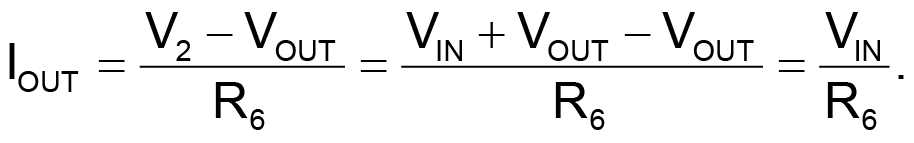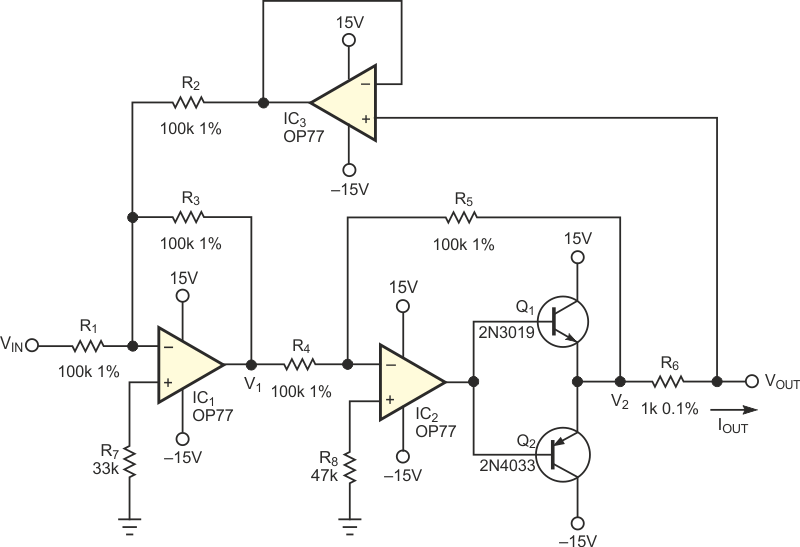# V/I converter accommodates grounded load

## Analog Devices OP77

Michele Frantisek

EDN

The voltage-to-current (V/I) converter in Figure 1 uses three common op amps, two medium-power transistors, and only a few passive components. The first op amp (IC1) inverts the sum of voltages VIN and VOUT to

V1 = –(VIN+VOUT).

The second op amp (IC2) and transistors Q1 and Q2 invert this voltage to produce

V2 = VIN + VOUT.

The formula for calculating the output current is thus:Figure 1. A versatile voltage-to-current converter provides a handy current source in many analog applications.

The formula shows that the value of IOUT depends only on VIN and R6. Voltage follower IC3 reduces to a negligible level the current from the circuit output to IC1. The advantages of the circuit are:

• Load-grounding possibility;
• Simple control of IOUT/VIN ratio;
• High precision, linearity, stability, and bandwidth;
• Wide IOUT range, approximately 1 µA to IC(max) of Q1 and Q2; and
• High output resistance of approximately 50 MΩ.

## Materials on the topic

EDNYou may have to register before you can post comments and get full access to forum.
 User Name Remember Me? Password